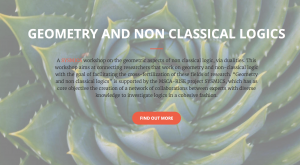## Corso di “Algebra della Logica” alla scuola AILA 2017This year I teach a course (12 hours) a the AILA summer school of logic.  Below one can find the slides of my first three lectures and some references.

• Lecture 1 (Classical propositional logic and Boolean algebras)
• Lecture 2 (Algebraic completeness of propositional calculus)
• Lecture 3 (Abstract Algebraic Logic)
• Lecture 4 (Dualities) lecture material:
• Lecture 5 and 6 (Non classical logic) references:
1. Y. Venema, Algebras and Coalgebras, in: J. van Benthem, P. Blackburn and F. Wolter (editors), Handbook of Modal Logic, 2006, pp 331-426.
2. R. L. O. Cignoli, I. M. L. D’Ottaviano e D. Mundici, Algebraic Foundations of Many-Valued Reasoning, Trends in Logic, Vol. 7 Springer, 2000.

Lecture notes by Guido Gherardi (Computability Theory).

## Workshop on “Geometry and non-classical logics”I am happy to announce that we will host a workshop on “Geometry and non-classical logics” at the campus of the University of Salerno, from the 5th to the 8th of September.

The workshop is one of the events planned within the EU-funded project SYSMICS.
Further information can be found at http://logica.dipmat.unisa.it/sysmics/geoNonLogic/

## The Twelfth International Tbilisi Symposium on Language, Logic and ComputationThe Twelfth International Tbilisi Symposium on Language, Logic and Computation will be held on 18-22 September 2017 in Kakheti, Georgia. The Programme Committee invites submissions for contributions on all aspects of language, logic and computation: https://easychair.org/conferences/?conf=tbillc2017. Submission deadline: 15 March 2017.

#### Tutorials:

Language: Jakub Szymanik (University of Amsterdam)
Logic: Sam van Gool (City College of New York)
Computation: Ana Sokolova (University of Salzburg)

#### Invited speakers:

Language:
Gemma Boleda (Universitat Pompeu Fabra)
Ruth Kempson (King’s College, London)
Logic:
Alexander Kurz (University of Leicester)
Eric Pacuit (University of Maryland)
Computation:
Dexter Kozen (Cornell University)
Alex Simpson (University of Ljubljana)

Further information at http://events.illc.uva.nl/Tbilisi/Tbilisi2017/

## Soft Computing Days

From the 23d to the 25th of May the Department of Mathematics will host “Soft Computing Days“, a bilateral workshop China-Italy .  We look forward to meeting you there.

## A(nother) duality for the whole variety of MV-algebras

This is the abstract of a talk I gave in Florence at Beyond 2014.

Given a category $C$ one can form its ind-completion by taking all formal directed colimits of objects in $C$. The “correct” arrows to consider are then families of some special equivalence classes of arrows in $C$ (Johnstone 1986, V.1.2, pag. 225). The pro-completion is formed dually by taking all formal directed limits. For general reasons, the ind-completion of a category $C$ is dually equivalent to the pro-completion of the dual category $C^{\rm op}$.

$$\textrm{ind}\mbox{-}C\simeq (\textrm{pro}\mbox{-}(C^{\rm{op}}))^{\rm{op}}. \qquad\qquad (1)$$

Ind- and pro- completions are very useful objects (as they are closed under directed (co)limits) but cumbersome to use, because of the involved definitions of arrows between objects. We prove that if $C$ is an algebraic category, then the situation considerably simplifies.

If $V$ is any variety of algebras, one can think of any algebra $A$ in $V$ as colimit of finitely presented algebras as follows.

Consider a presentation of $A$ i.e., a cardinal $\mu$ and a congruence [/latex]\theta[/latex] on the free $\mu$-generated algebra $\mathcal{F}(\mu)$ such that $A\cong \mathcal{F}(\mu)/\theta$. Now, consider the set $F(\theta)$ of all finitely generated congruences contained in $\theta$, this gives a directed diagram in which the objects are the finitely presented algebras of the form $\mathcal{F}(n)/\theta_{i}$ where $\theta_{i}\in F(\theta)$ and $X_{1},...,X_{n}$ are the free generators occurring in $\theta_{i}$. It is straightforward to see that this diagram is directed, for if $\mathcal{F}(m)/\theta_{1}$ and $\mathcal{F}(n)/\theta_{2}$ are in the diagram, then both map into $\mathcal{F}(m+n)/\langle\theta_{1}\uplus\theta_{2}\rangle$, where $\langle\theta_{1}\uplus\theta_{2}\rangle$ is the congruence generated by the disjoint union of $\theta_{1}$ and $\theta_{2}$. Now, the colimit of such a diagram is exactly $A$.

Denoting by $V_{\textrm{fp}}$ the full subcategory of $V$ of finitely presented objects, the above reasoning entails

$$V\simeq\textrm{ind}\mbox{-}V_{\textrm{fp}}. \qquad\qquad (2)$$

We apply our result to the special case where $V$ is the class of MV-algebras. One can then combine the duality between finitely presented MV-algebras and the category $P_{\mathbb{Z}}$ of rational polyhedra with $\mathbb{Z}$-maps (see here), with (1)  and (2) to obtain,

$$MV\simeq\textrm{ind}\mbox{-}MV_{\textrm{fp}}\simeq \textrm{pro}\mbox{-}(P_{\mathbb{Z}})^{\rm{op}}. \qquad\qquad (3)$$

This gives a categorical duality for the whole class of MV-algebras whose geometric content may be more transparent than other dualities in literature. In increasing order of complexity one has that any MV-algebra:

1. is dual to a polyhedron (Finitely presented case);
2. is dual to an intersection of polyhedra (Semisimple case);
3. is dual to a countable nested sequence of polyhedra (Finitely generated case);
4. is dual to the directed limit of a family of polyhedra. (General case).

Here are the slides of this talk